# Python Matplotlib drawing 3D graphics

2022-02-01 02:15:08This is my participation 11 The fourth of the yuegengwen challenge 19 God , Check out the activity details ：2021 One last more challenge

# Preface

stay matplotlib At first, the module only supported drawing 2D graphics , For example, we have also learned to draw a line chart before 、 Histogram 、 Histogram and other statistical charts , At the same time, learn to draw multiple subgraphs 、 Common graphics, etc . Here are some examples of previous articles , For reference

With the development of technology ,matplotlib Modules in 2D The basis of drawing , Encapsulate practical 3D Drawing toolkit mplot3d To support us to draw faster 3D graphics .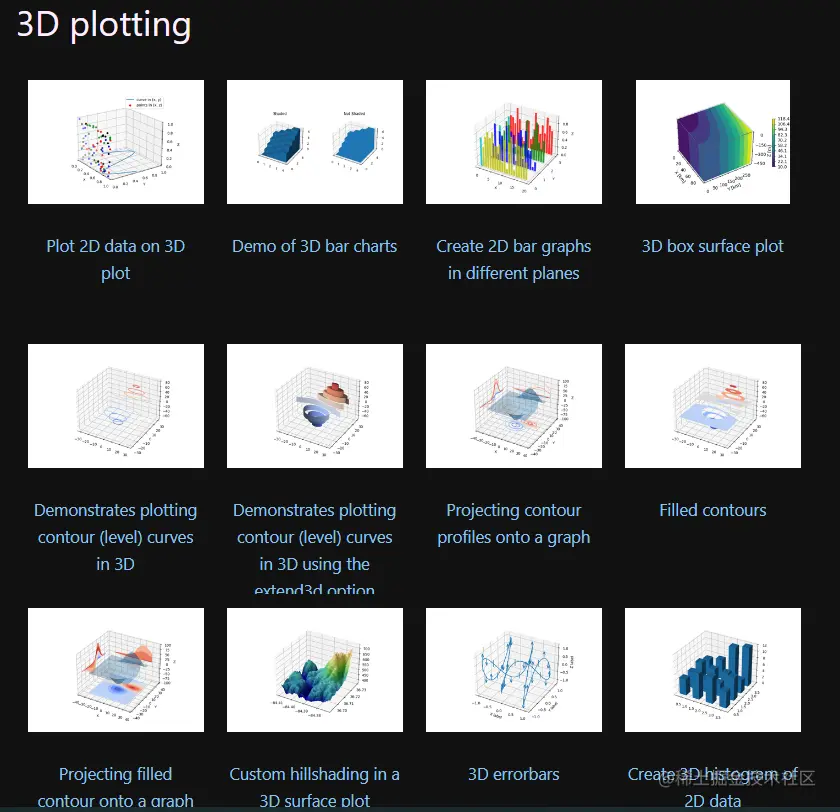In this issue , We will study in detail 3D Drawing module mplot3d Related drawing methods ,Let's go~

# 1. mplot3d summary

mplot3d Kuo is matplotlib The module is dedicated to drawing 3D The image provides 2D Projective Axes object , by Matplotlib Added the ability to plot scatter plot 、 Surface graph 、 Broken line diagram 、 Grid, etc .

• mplot3d characteristic

• mplot3d Allow users to create matplotlib 2D Drawing is simple 3D graphics
• mplot3d The method is easy to use , Directly available 2D Method to draw
• mplot3d draw 3D Image and 2D Images share the same rendering engine , There may be Z Deviation of axis projection
• mplot3d Styles can be personalized by changing parameters
• mplot3d Use

We are using mplot3d When it comes to toolkits , Need extra use from...import Import Axes3D class

``````from  mpl_toolkits.mplot3d import Axes3D
Copy code ``````

PS：

• pyplot Class cannot 3D Add content to the drawing 、 Handle 3D Additional information, etc , You have to use Axes3D Object to create
• mplot3d draw 3D Graphical methods are not yet mature , For complex 3D Figure scene , It is recommended to use Mayavi

# 2. draw 3D Graphic steps

stay matplotlib Drawing is provided in the module 3D Graphics need mplot3d in Axes3D Object and the pyplot Methods used in combination , So draw 3D There are the following steps for graphics

• Import matplotlib.pyplot and mpl_toolkits.mplot3d in Axes3D class
``````import matplotlib.pyplot as plt
from  mpl_toolkits.mplot3d import Axes3D
Copy code ``````
• Use Pyplot.figure Create a fig Canvas object
``````fig = plt.figure()
Copy code ``````
• stay fig Create a band in the canvas 3D Coordinate Axes object

• Mode one ： Use to create subgraphs through projection='3D' To create
``````ax = fig.add_subplot(projection='3d')
Copy code ``````
• Mode two ： call Axes3D Class to create objects
``````ax = Axes3D(fig)
Copy code ``````
• call numpy.random perhaps numpy.arange() And so on x,y Axis data

``````x = np.arange(-5,5,0.25)
y = np.arange(-5,5,0.25)
Copy code ``````
• call numpy.meshgrid() Method pair x,y Mapping matrix
``````x,y = np.meshgrid(x,y)
Copy code ``````
• according to x,y The matrix data is calculated according to the demand Z Axis data , For example, call numpy.sin()、cos() function
``````R = np.sqrt(x**2+y**2)
z = np.cos(R)
Copy code ``````
• Axes Object call pyplot Charting 、 Drawing by graphic method , For example, call contour map contour、 Surfaces plot_surface()
``````ax.plot_surface(x,y,z,rstride=1,cstride=1,alpha=0.5,cmap=cm.coolwarm)

ax.contour(x,y,z,zdir='z',offset=-2)
Copy code ``````
• Axes Object call xlim,ylim,zlim Method setting x,y,z Range of axis values
``````ax.set_zlim(-2,2)
Copy code ``````
• Last call pyplot.show() Show the drawn image
``````plt.show()
Copy code ``````
• The effect of the display is shown in the figure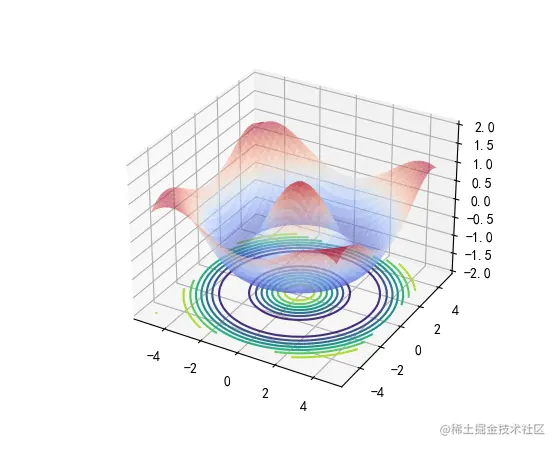# 3. draw 3D Scatter plot

• Use numpy.arange()|numpy.random.randint() Get ready x,y,z Axis of the data
• Axes Object call scatter Scatter plot method to draw scatter plot
``````x = np.arange(0,200)
y = np.arange(0,100)

x,y = np.meshgrid(x,y)

z = np.random.randint(0,200,size=(100,200))

yc = np.arctan2(x,y)

ax.scatter(x,y,z,c=yc,s=50,marker=".")
Copy code ``````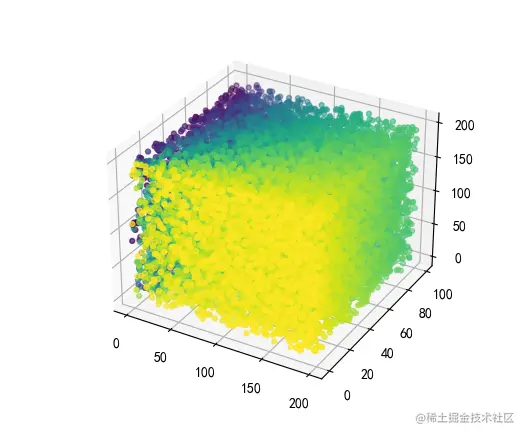# 4. draw 3D Broken line diagram

• Use np.linspace() and np.sin() Yes x,y Axis data preparation
• Axes Object call plot() Draw a line chart with the line method
``````x = np.linspace(0,1,100)
y = np.sin(x*2*np.pi)/2+0.5
ax.plot(x,y,zs=0,zdir="z")
Copy code ``````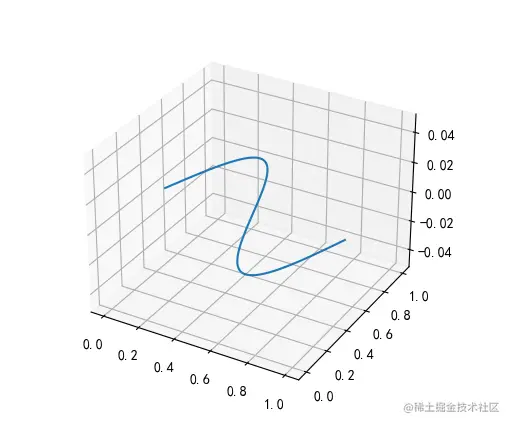# 5. draw 3D Histogram

``````for z in range(0,3):

x = np.arange(1,13)
y = 1000*np.random.rand(12)
color = plt.cm.Set2(random.choice(range(plt.cm.Set2.N)))
ax.bar(x,y,zs=z,zdir="y",color=color,alpha=0.8)

ax.set_xlabel("x")
ax.set_ylabel("y")
ax.set_zlabel("z")
Copy code ``````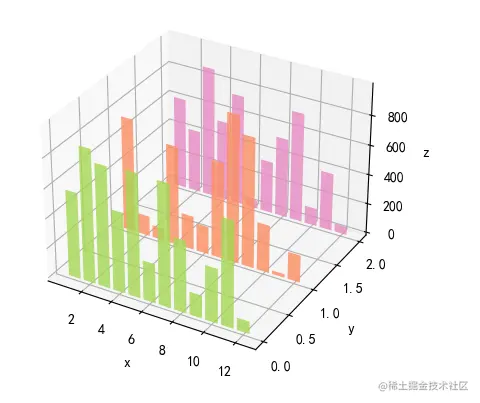# summary

In this issue , Yes matplotlib Module supply mplot3d Library drawing 3D Learn the steps of graphics , At the same time, manually operate the scatter diagram 、 Broken line 、 Histogram 3D chart .

about , draw 3D Graphics must now use Axes3D Object to draw , For complex 3D graphics matplotlib The rendering engine renders poorly , Official recommended use Mayavi To deal with .

The above is the content of this issue , Welcome big guys to praise and comment , See you next time ~